# HSPT Math : How to solve two-step equations

## Example Questions

1 2 3 4 5 6 7 9 Next →

### Example Question #81 : Common Core Math: Grade 5

Solve: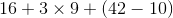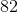Explanation:When solving this problem, remember order of operations PEMDAS. The parentheses come first, followed by the multiplication, and then addition.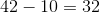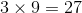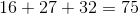### Example Question #1 : Solve Word Problems Leading To Equations: Ccss.Math.Content.7.Ee.B.4a

Mark is three times as old as his son Brian. In ten years, Mark will be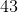years old. In how many years will Mark be twice as old as Brian?Explanation:

In ten years, Mark will beyears old, so Mark is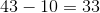years old now, and Brian is one-third of this, or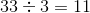years old.

Letbe the number of years in which Mark will be twice Brian's age. Then Brian will be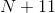, and Mark will be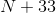. Since Mark will be twice Brian's age, we can set up and solve the equation: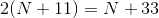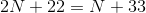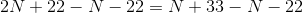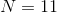Mark will be twice Brian's age inyears.

### Example Question #81 : How To Solve Two Step Equations

Gary is twice as old as his niece Candy. How old will Candy will be in five years when Gary is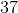years old?Not enough information is given to determine the answer.Explanation:

Since Gary will be 37 in five years, he is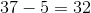years old now. He is twice as old as Cathy, so she is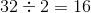years old, and in five years, she will be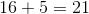years old.

### Example Question #31 : Solve Word Problems Leading To Inequalities: Ccss.Math.Content.7.Ee.B.4b

A parking garage charges a minimum fee of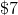to park in the garage. It also charges an additional fee of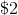for every hour, or portion of an hour, in which a person parks in the garage. Cliff wants to pay a maximum of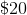to park there. If he pulls into the garage at exactly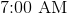, when must he leave at latest?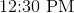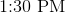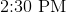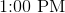Explanation:

Letbe the number of hours that Cliff parks his car in the garage. Since he pays a flat fee ofplusper hour or portion thereof, he pays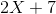dollars. Since he does not want to spend more than,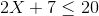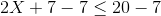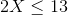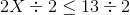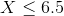Since a portion of an hour counts as much as an hour,must be a whole number.implies that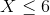.

Cliff can park in the garage for a maximum of 6 hours, and since he entered the garage at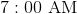, he must exithours later: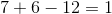,

or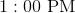.

### Example Question #82 : How To Solve Two Step Equations

Solve the following: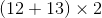Explanation:When solving this problem, remember order of operations PEMDAS. The parentheses come first followed by the multiplication.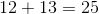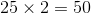1 2 3 4 5 6 7 9 Next →

### All HSPT Math Resources